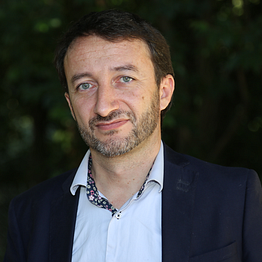# Introduction

In the domain of software development, the Cognitive Complexity could be approximately defined as “the difficulty for a human being to understand some code snippet”. This definition seems to be clear and without ambiguities, but it is not.

The main problem is in the word “difficulty”: what does it mean and how to measure it ? That’s why, in this article, I will use a more precise definition, which uses a notion which could be measured in the real world: the time.

Definition
The Cognitive Complexity of a code snippet is the time required by a mean developer to understand it…

# Do you really understand the code that you read ?

If you tell me that you understood some code snippet, how could I be sure of it ? What should I check ? Even if the code to read is a simple function returning a number, without any side effects, how could you prove to me that you really understood it ?

If you reply “That’s simple: I understand a function `f` if I am able to predict the value of the number returned by `f`”, I’m sorry, but you are wrong :

`function random(): number {  return Math.random();}`

Are you able to predict the value returned by the…

# Introduction

The cognitive complexity of a code snippet is one of the main parameters affecting the maintainability of a software. An approximative definition of the cognitive complexity could be “the difficulty for a developer to understand a code snippet”. Unfortunately, this definition is too vague to be able to measure it, and there is a lack of scientific consensus of what is precisely the cognitive complexity. This fact implies that many approaching definitions exist, thus many formulas to measure it, and finally different results for the same intuitive notion.

In a previous article, I gave the definition below:

Definition
The cognitive complexity…## Gilles Fabre

TypeScript expert. Specialization in static code analysis and in software complexity